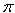Subject: calculating the radius of a hemisphere Name: Emma Who are you: Student how do you calculate the radius of a hemisphere when you are given the volume? thanks Hi Emma. A sphere's volume is V = 4/3r3. A hemisphere is half a sphere. Can you take it from here? Stephen La Rocque.